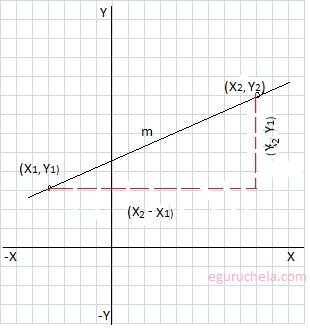#### Find the Slope, Distance, Angle and Equation of Line

Online calculator to find slope, length/distance, angle and equation of a line segment for entered the coordinates (x1, y1), (x2, y2) of two points, using following formula :
m = (y2-y1) / (x2-x1) and Distance = √ ( (x2-x1)2 + (y2-y1)2 )
Angle = arctan ( m ) and
Line of Equation is y = mx + b , Where m = slopex1 = y1 = x2 = y2 = Slope       : Distance  : Angle      : Equation :
Let's take the example of line passing through
two ponits coordinates are (-4, 3) and (9, 8):
$$\text{find the slop } m = \frac{(y_2 - y_1)}{(x_2 - x_1)}$$ $$m = \frac{(8 - 3)}{(9 - (-4))}$$ $$m = \frac{(5)}{(9 + 4)}$$ $$m = \frac{5}{13}$$ $$m = 0.3846153846153846$$ $$m = 0.3846$$
Now find the distance using √ ( (x2-x1)2 + (y2-y1)2 ) $$distance = \sqrt { (x_2 - x_1)^2 + (y_2 - y_1)^2}$$ $$= \sqrt { (9 - (-4))^2 + (8 - 3)^2}$$ $$= \sqrt { (9 + 4)^2 +(8 - 3)^2}$$ $$= \sqrt { (13)^2 + (5)^2}$$ $$= \sqrt {169+ 25}$$ $$= \sqrt {194}$$ $$= 13.928$$
The slop is defined as opposite/adjacent and in trigonometry tan θ = opposite/adjacent, so we can say $$\tan \theta = \frac{opposite}{adjacent} = m$$ $$\tan \theta = m$$ we can find the angle θ using: $$\theta = \arctan(m)$$ $$\theta = \arctan(0.3846)$$ $$\theta = 21.0367$$ This angle is also called inclination of line.
Now we can find a linear equation using following formula:
$$y = mx + b$$ Now we can calculate b. as we already know the slope (0.3846)
The equation can be re-written as:
$$b = y -mx$$ We will take one of the given points (-4, 3) and apply the values in formula.
$$b = 3 -0.3846 \times (-4)$$ $$b = 3 + 1.5384 = 4.5384$$ We can use the other point (9,8) to check the value of b: $$b = 8 -0.3846 \times (9)$$ $$b = 8 - 3.4614 = 4.5386$$ Now apply the values to the equation : $$y = mx + b$$ $$y = 0.3846 x + 4.5386$$